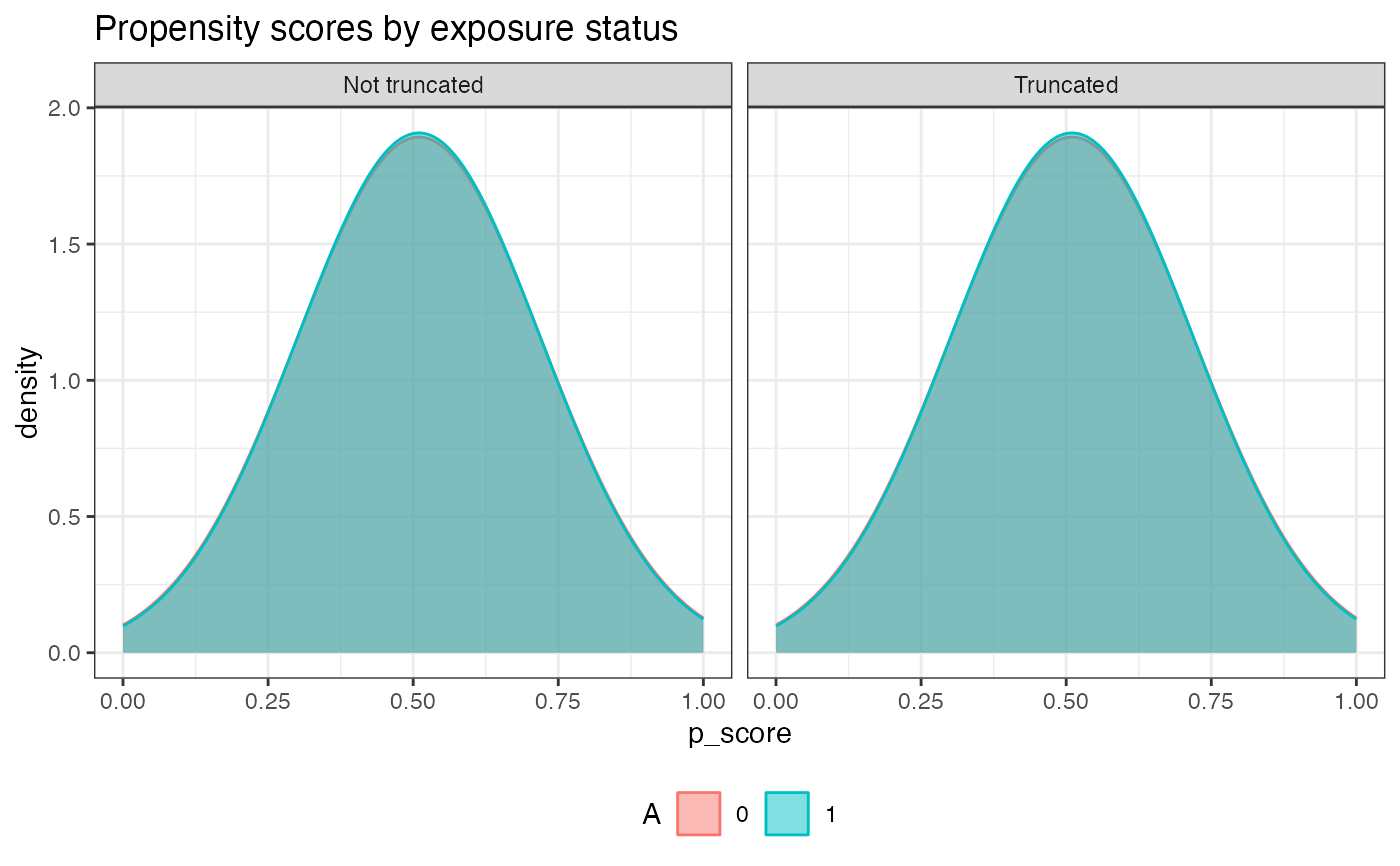An R6Class of AIPW for estimating the average causal effects with users' inputs of exposure, outcome, covariates and related libraries for estimating the efficient influence function.

## Value

AIPW object

## Details

An AIPW object is constructed by new() with users' inputs of data and causal structures, then it fit() the data using the libraries in Q.SL.library and g.SL.library with k_split cross-fitting, and provides results via the summary() method. After using fit() and/or summary() methods, propensity scores and inverse probability weights by exposure status can be examined with plot.p_score() and plot.ip_weights(), respectively.

If outcome is missing, analysis assumes missing at random (MAR) by estimating propensity scores of I(A=a, observed=1) with all covariates W. (W.Q and W.g are disabled.) Missing exposure is not supported.

See examples for illustration.

## Constructor

AIPW$new(Y = NULL, A = NULL, W = NULL, W.Q = NULL, W.g = NULL, Q.SL.library = NULL, g.SL.library = NULL, k_split = 10, verbose = TRUE, save.sl.fit = FALSE) ### Constructor Arguments  Argument Type Details Y Integer A vector of outcome (binary (0, 1) or continuous) A Integer A vector of binary exposure (0 or 1) W Data Covariates for both exposure and outcome models. W.Q Data Covariates for the outcome model (Q). W.g Data Covariates for the exposure model (g). Q.SL.library SL.library Algorithms used for the outcome model (Q). g.SL.library SL.library Algorithms used for the exposure model (g). k_split Integer Number of folds for splitting (Default = 10). verbose Logical Whether to print the result (Default = TRUE) save.sl.fit Logical Whether to save Q.fit and g.fit (Default = FALSE) ### Constructor Argument Details W, W.Q & W.g It can be a vector, matrix or data.frame. If and only if W == NULL, W would be replaced by W.Q and W.g. Q.SL.library & g.SL.library Machine learning algorithms from SuperLearner libraries or sl3 learner object (Lrnr_base) k_split It ranges from 1 to number of observation-1. If k_split=1, no cross-fitting; if k_split>=2, cross-fitting is used (e.g., k_split=10, use 9/10 of the data to estimate and the remaining 1/10 leftover to predict). NOTE: it's recommended to use cross-fitting. save.sl.fit This option allows users to save the fitted sl object (libs$Q.fit & libs$g.fit) for debug use. Warning: Saving the SuperLearner fitted object may cause a substantive storage/memory use. ## Public Methods  Methods Details Link fit() Fit the data to the AIPW object fit.AIPW stratified_fit() Fit the data to the AIPW object stratified by A stratified_fit.AIPW summary() Summary of the average treatment effects from AIPW summary.AIPW_base plot.p_score() Plot the propensity scores by exposure status plot.p_score plot.ip_weights() Plot the inverse probability weights using truncated propensity scores plot.ip_weights ## Public Variables  Variable Generated by Return n Constructor Number of observations stratified_fitted stratified_fit() Fit the outcome model stratified by exposure status obs_est fit() & summary() Components calculating average causal effects estimates summary() A list of Risk difference, risk ratio, odds ratio result summary() A matrix contains RD, ATT, ATC, RR and OR with their SE and 95%CI g.plot plot.p_score() A density plot of propensity scores by exposure status ip_weights.plot plot.ip_weights() A box plot of inverse probability weights libs fit() SuperLearner or sl3 libraries and their fitted objects sl.fit Constructor A wrapper function for fitting SuperLearner or sl3 sl.predict Constructor A wrapper function using sl.fit to predict ### Public Variable Details stratified_fit An indicator for whether the outcome model is fitted stratified by exposure status in thefit() method. Only when using stratified_fit() to turn on stratified_fit = TRUE, summary outputs average treatment effects among the treated and the controls. obs_est After using fit() and summary() methods, this list contains the propensity scores (p_score), counterfactual predictions (mu, mu1 & mu0) and efficient influence functions (aipw_eif1 & aipw_eif0) for later average treatment effect calculations. g.plot This plot is generated by ggplot2::geom_density ip_weights.plot This plot uses truncated propensity scores stratified by exposure status (ggplot2::geom_boxplot) Zhong Y, Kennedy EH, Bodnar LM, Naimi AI (2021). AIPW: An R Package for Augmented Inverse Probability Weighted Estimation of Average Causal Effects. American Journal of Epidemiology. Robins JM, Rotnitzky A (1995). Semiparametric efficiency in multivariate regression models with missing data. Journal of the American Statistical Association. Chernozhukov V, Chetverikov V, Demirer M, et al (2018). Double/debiased machine learning for treatment and structural parameters. The Econometrics Journal. Kennedy EH, Sjolander A, Small DS (2015). Semiparametric causal inference in matched cohort studies. Biometrika. ## Examples library(SuperLearner) #> Loading required package: nnls#> Loading required package: gam#> Loading required package: splines#> Loading required package: foreach#> Loaded gam 1.20#> Super Learner#> Version: 2.0-28#> Package created on 2021-05-04library(ggplot2) #create an object aipw_sl <- AIPW$new(Y=rbinom(100,1,0.5), A=rbinom(100,1,0.5),
W.Q=rbinom(100,1,0.5), W.g=rbinom(100,1,0.5),
Q.SL.library="SL.mean",g.SL.library="SL.mean",
k_split=1,verbose=FALSE)

#fit the object
aipw_sl$fit() # or use aipw_sl$stratified_fit() to estimate ATE and ATT/ATC

#calculate the results
aipw_sl$summary(g.bound = 0.025) #check the propensity scores by exposure status after truncation aipw_sl$plot.p_score()